用乐高积木给你的宝宝讲懂贝叶斯定理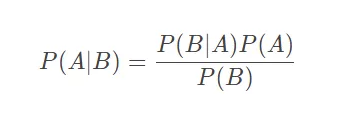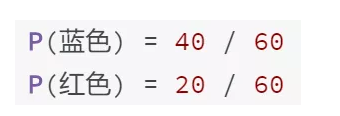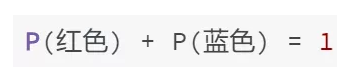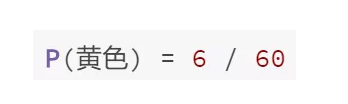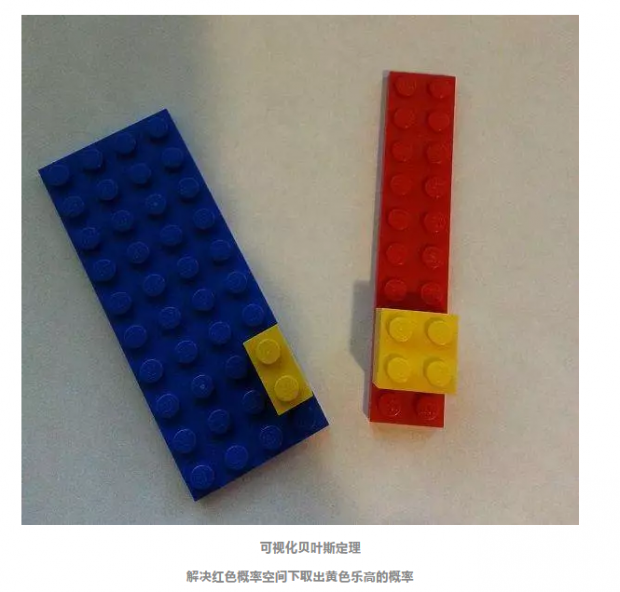1. 将红色积木和蓝色积木分开

2. 我们得到面积为2*10的红色概率空间

3. 在这个空间上，黄色积木有4块

4. 将黄色积木和红色积木分开

5. 计算出P(黄色 | 红色) = 4/20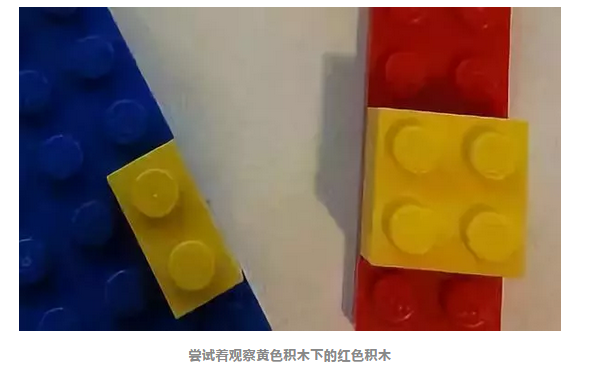红色积木的个数 = P(红色) * 总积木的数量 = 1/3*60 = 20

P(黄色|红色) * 红色积木的个数 = 1/5*20 = 4

1. 首先我们试着用数学的语言，推出“一共有6块黄色积木”这个结论。推出这个结论，我们只需要用通过以下计算：

黄色积木的个数  =  P(黄色) * 总积木的数量 = 1/10*60 = 6

2. 接着，如何得到“其中4块底下是红色”这个结论呢？

3. 最后计算出红色积木上是黄色积木的概率：

P(红色|黄色) = 在黄色下面的红色积木的个数 /  黄色积木的个数 = 4/6

P(红色|黄色)  = P(黄色|红色) * 红色积木的个数  /  P(黄色) * 总积木的数量

P(红色|黄色)  = P(黄色|红色)* P(红色) * 总积木的数量  / P(黄色) * 总积木的数量

P(红色|黄色)  = P(黄色|红色)* P(红色)  / P(黄色）

• 概念上，贝叶斯定理是服从直觉的。（我们只是用数学语言重写了一遍我们的直觉的推理。）

• 同时，贝叶斯定理的形式化表示，不一定是显而易见的。（推出最终的公式，我们还需要多几步数学的表达）

• Learn about Bayesian Priors with Han Solo

https://www.countbayesie.com/blog/2015/2/18/hans-solo-and-bayesian-priors

• Understand Bayes' Factor and Bayesian Reasoning by exploring a classic episode of the Twilight Zone

https://www.countbayesie.com/blog/2016/3/16/bayesian-reasoning-in-the-twilight-zone

• Use Bayes' Theorem to reason about the probability that your friends are really allergic to gluten